Ex 7.3

Chapter 7 Class 8 Comparing Quantities
Serial order wise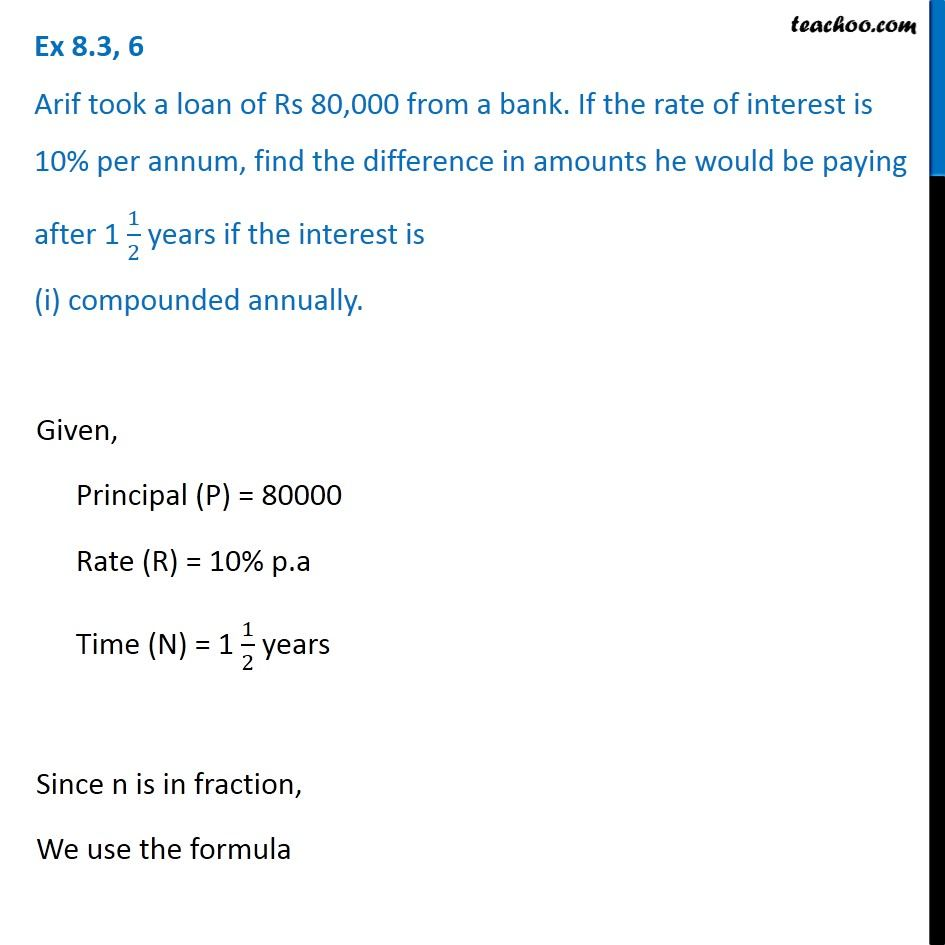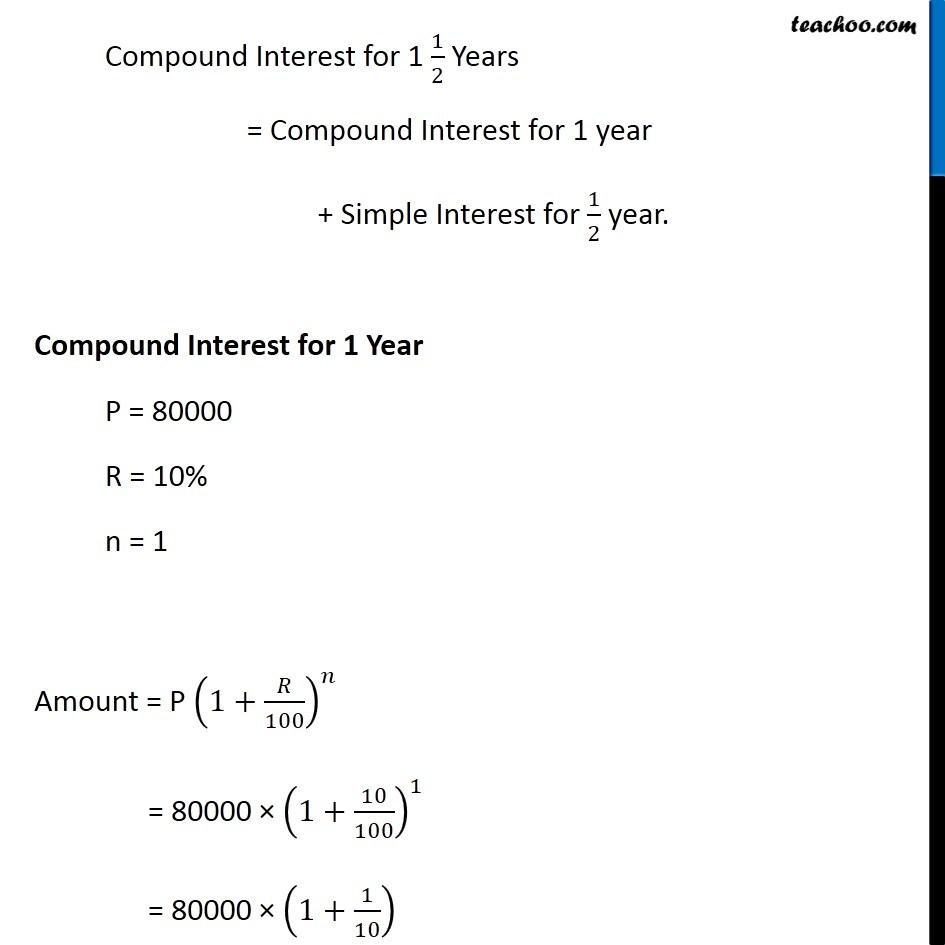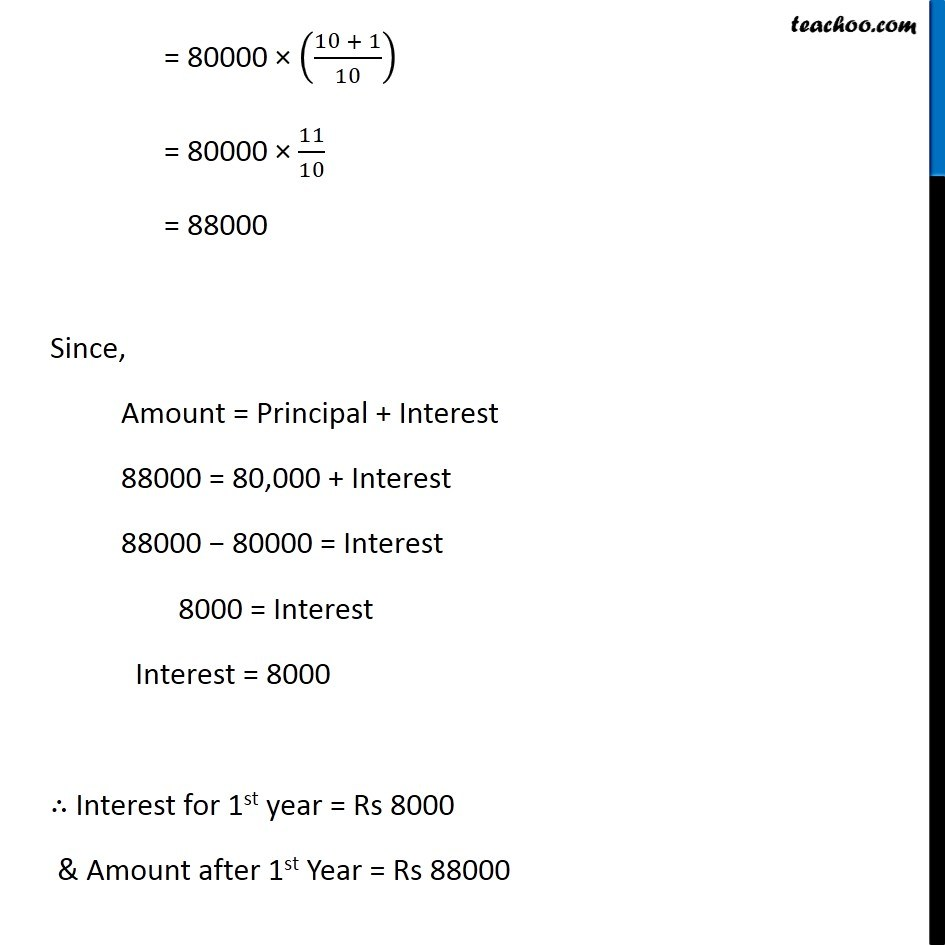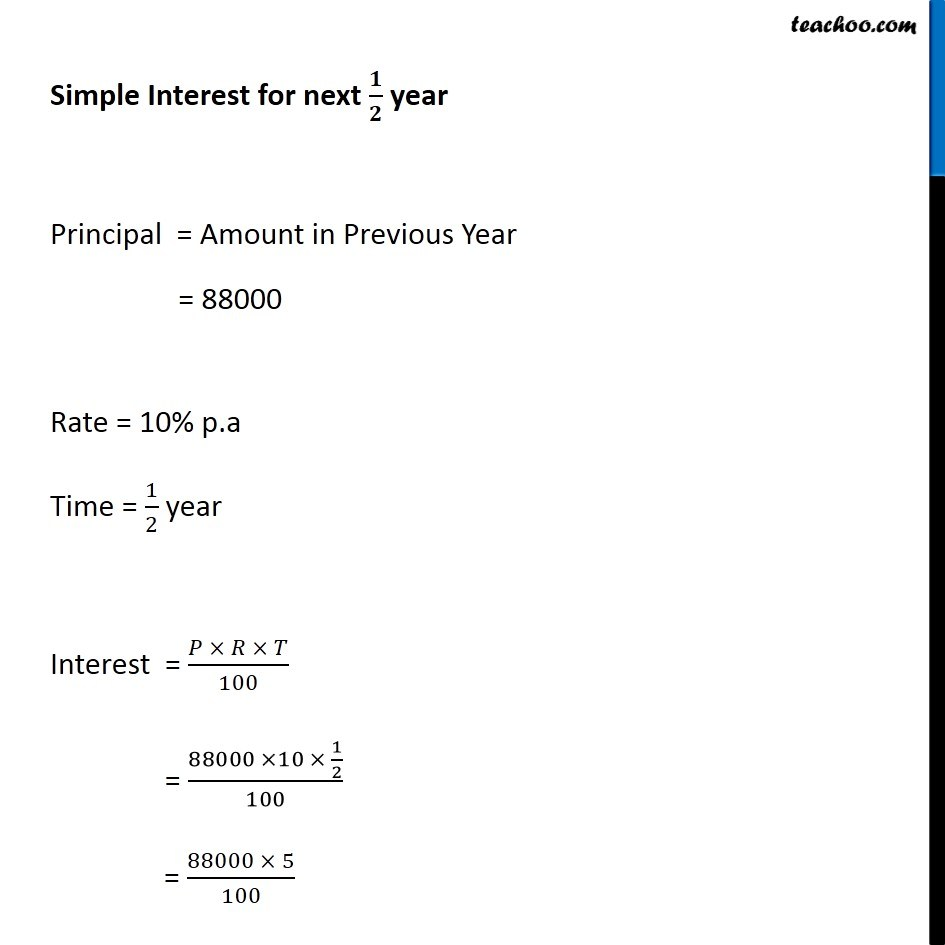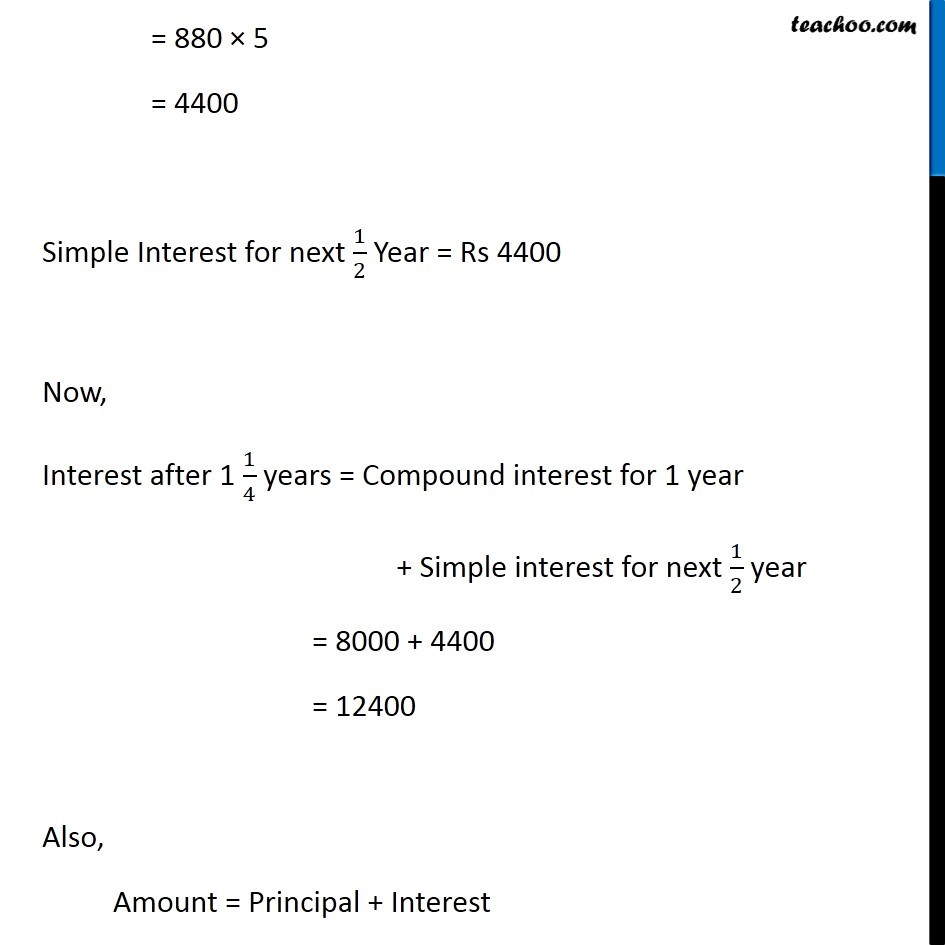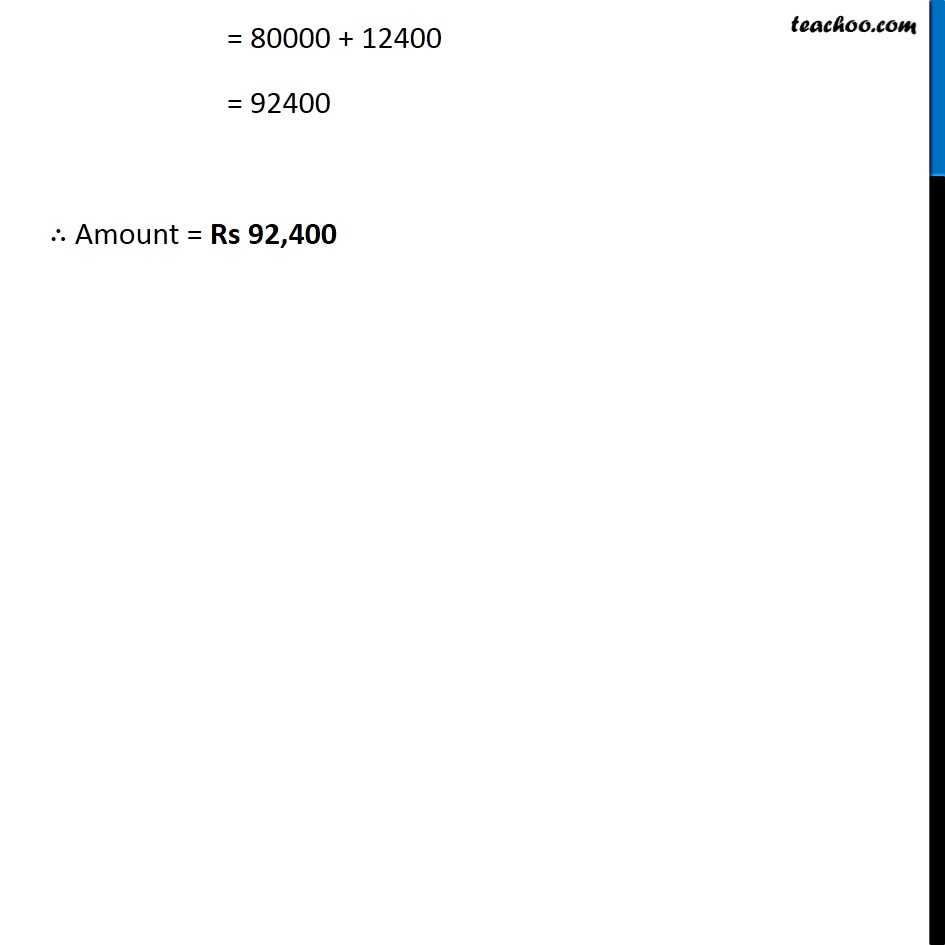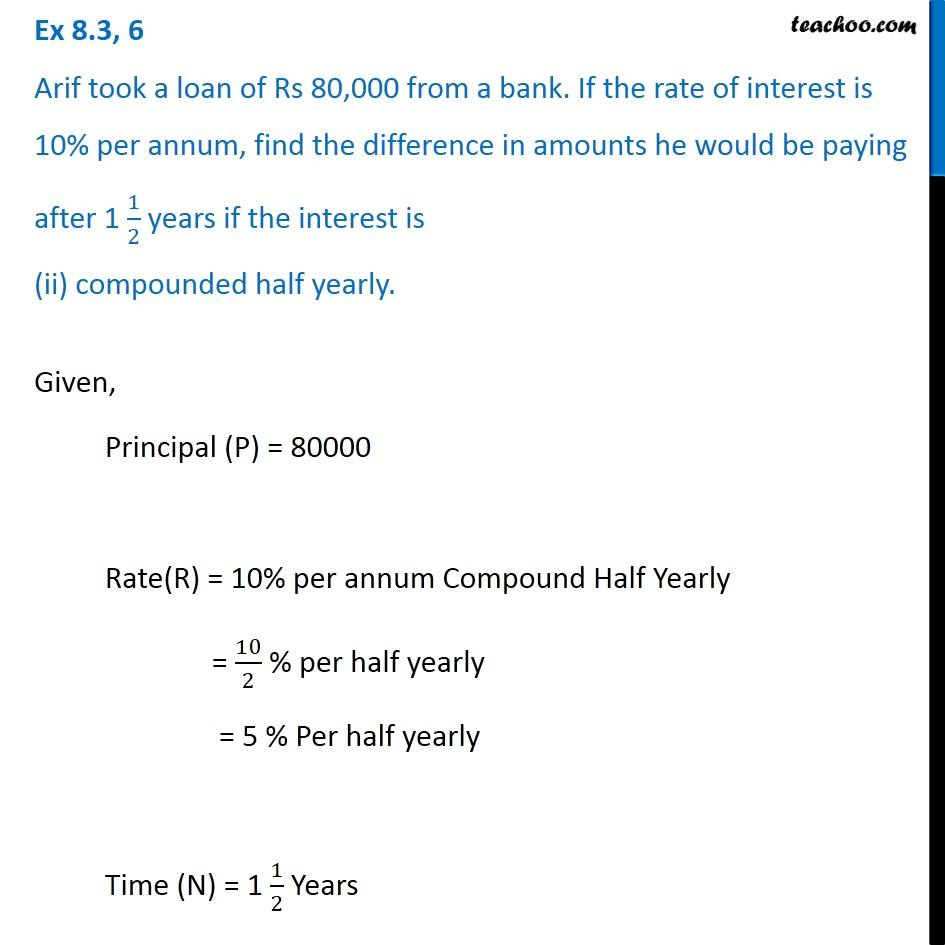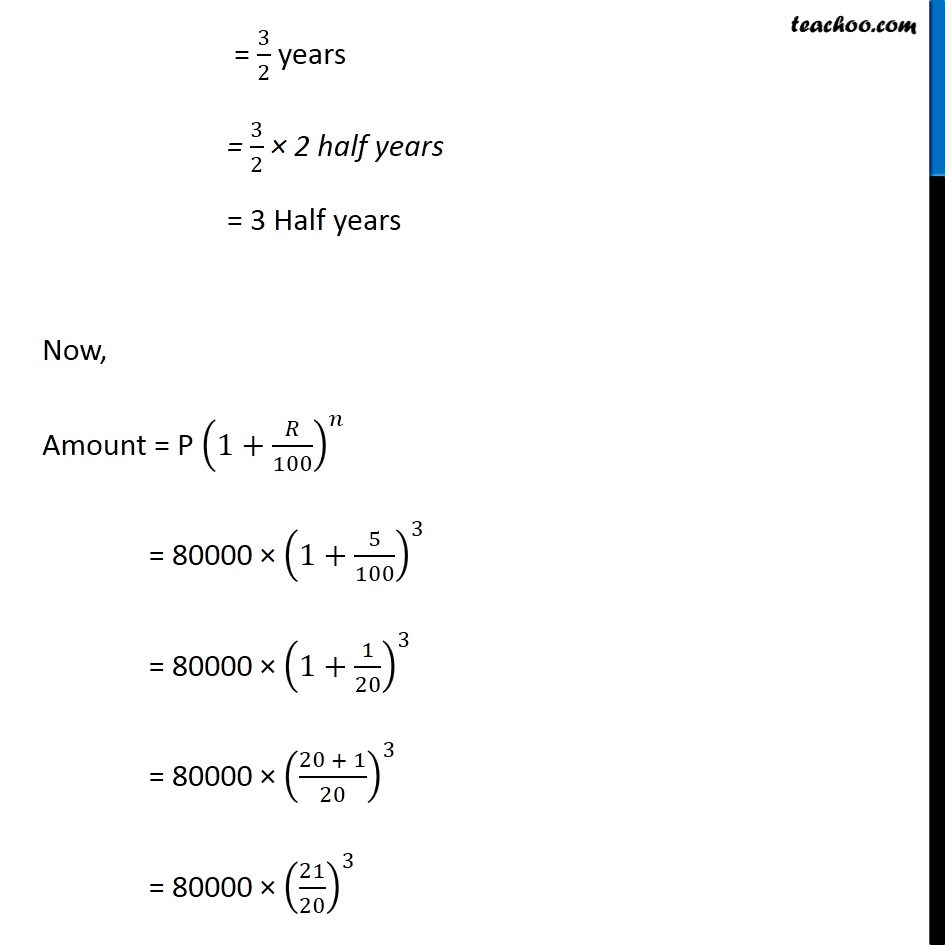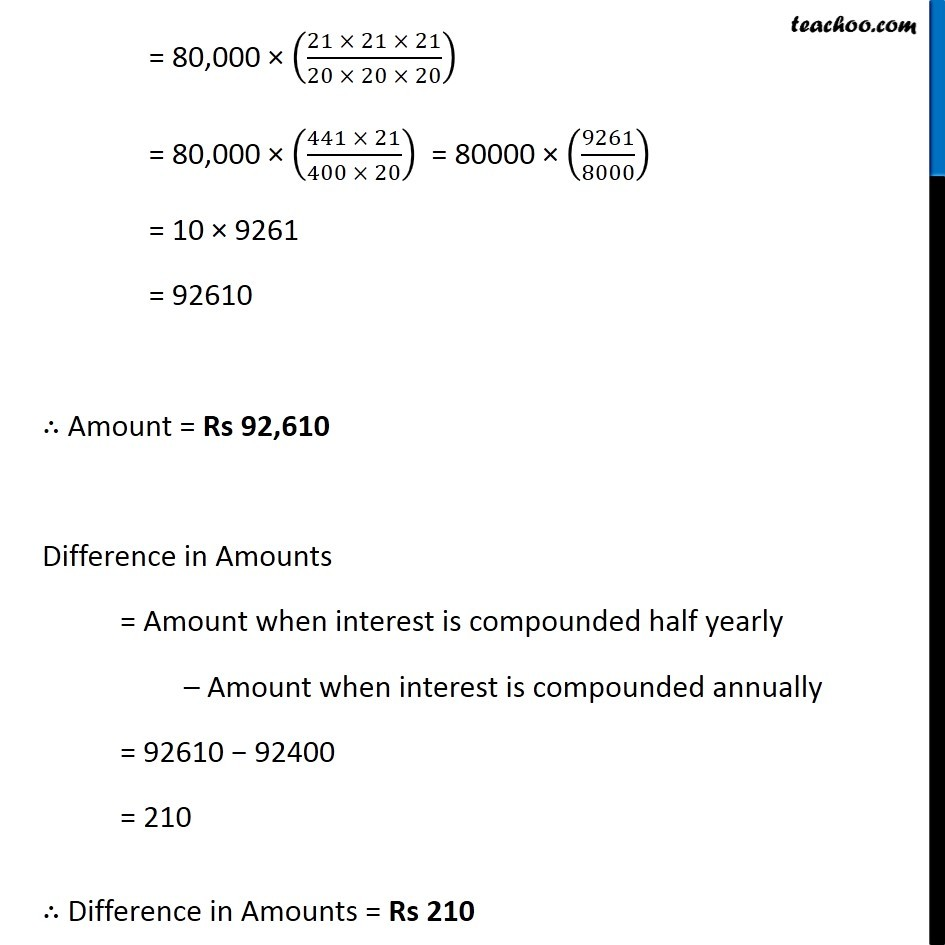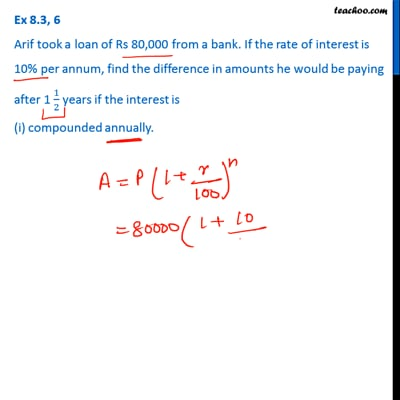This video is only available for Teachoo black users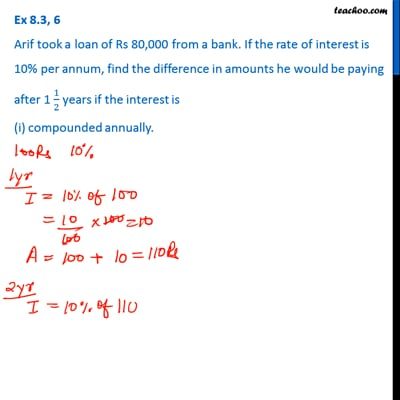This video is only available for Teachoo black users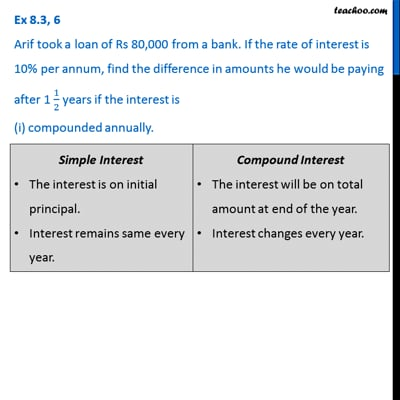This video is only available for Teachoo black users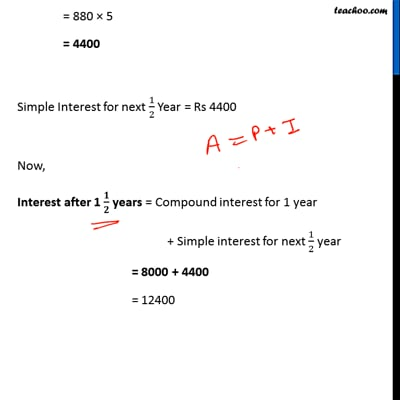This video is only available for Teachoo black users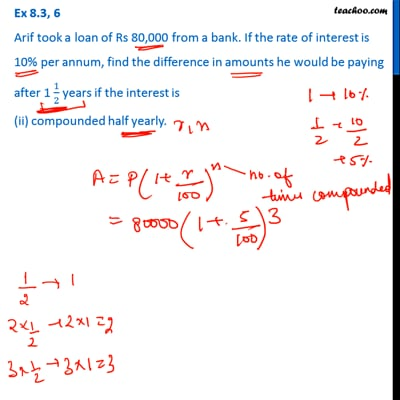This video is only available for Teachoo black users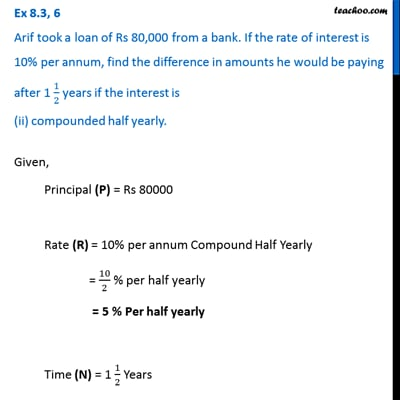This video is only available for Teachoo black users

Learn in your speed, with individual attention - Teachoo Maths 1-on-1 Class

### Transcript

Question 6 Arif took a loan of Rs 80,000 from a bank. If the rate of interest is 10% per annum, find the difference in amounts he would be paying after 1 1/2 years if the interest is (i) compounded annually. Given, Principal (P) = 80000 Rate (R) = 10% p.a Time (N) = 1 1/2 years Since n is in fraction, We use the formula Compound Interest for 1 1/2 Years = Compound Interest for 1 year + Simple Interest for 1/2 year. Compound Interest for 1 Year P = 80000 R = 10% n = 1 Amount = P (1+𝑅/100)^𝑛 = 80000 × (1+10/100)^1 = 80000 × (1+1/10) = 80000 × ((10 + 1)/10) = 80000 × 11/10 = 88000 Since, Amount = Principal + Interest 88000 = 80,000 + Interest 88000 − 80000 = Interest 8000 = Interest Interest = 8000 ∴ Interest for 1st year = Rs 8000 & Amount after 1st Year = Rs 88000 Simple Interest for next 𝟏/𝟐 year Principal = Amount in Previous Year = 88000 Rate = 10% p.a Time = 1/2 year Interest = (𝑃 × 𝑅 × 𝑇)/100 = (88000 ×10 × 1/2)/100 = (88000 × 5)/100 = 880 × 5 = 4400 Simple Interest for next 1/2 Year = Rs 4400 Now, Interest after 1 1/4 years = Compound interest for 1 year + Simple interest for next 1/2 year = 8000 + 4400 = 12400 Also, Amount = Principal + Interest = 80000 + 12400 = 92400 ∴ Amount = Rs 92,400 Question 6 Arif took a loan of Rs 80,000 from a bank. If the rate of interest is 10% per annum, find the difference in amounts he would be paying after 1 1/2 years if the interest is (ii) compounded half yearly. Given, Principal (P) = 80000 Rate(R) = 10% per annum Compound Half Yearly = 10/2 % per half yearly = 5 % Per half yearly Time (N) = 1 1/2 Years = 3/2 years = 3/2 × 2 half years = 3 Half years Now, Amount = P (1+𝑅/100)^𝑛 = 80000 × (1+5/100)^3 = 80000 × (1+1/20)^3 = 80000 × ((20 + 1)/20)^3 = 80000 × (21/20)^3 = 80,000 × ((21 × 21 × 21)/(20 × 20 × 20)) = 80,000 × ((441 × 21)/(400 × 20)) = 80000 × (9261/8000) = 10 × 9261 = 92610 ∴ Amount = Rs 92,610 Difference in Amounts = Amount when interest is compounded half yearly – Amount when interest is compounded annually = 92610 − 92400 = 210 ∴ Difference in Amounts = Rs 210